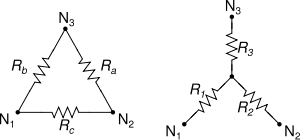# Resistor series proof and the Y-Δ transform

Bipolarity
If you have resistors in series ---\/\/\/\/---\/\/\/\/\/--- then the equivalent resistance of this system will merely be the sum of the individual resistors in the series. But the proof of this result is based on the fundamental assumption that the currents going through the two resistors (as well as through the equivalent resistor) are identical.

In the derivations for the equations of the Y-Δ transform, as shown in the picture, the idea is that ## R_{c} = R_{1} + R_{2} ##. This follows from the fact that R1 and R2 are in "series", but they technically aren't since there is a branch coming out between them going to R3. They can only be in series if the current going through them is identical (this is the definition of series), but this implies that the current going through R3 is 0, which may not always be true!

So what's the deal with this? It's been bothering me for a while as virtually no one has been able to explain this. Some people have said that for the sake of circuit analysis, the branch where R3 is located is totally ignored for the sake of evaluating ##R_{c}##, but I see no justification for this.

So either something is wrong with my understanding of the Y-Δ transform or something is wrong with my understanding of how series/parallel resistors really work. Which is it and why?

BiP

eigenperson
In the derivations for the equations of the Y-Δ transform, as shown in the picture <snip>, the idea is that ## R_{c} = R_{1} + R_{2} ##.
I don't believe this is the idea at all. In fact $$R_c = {R_1R_2 + R_2R_3 + R_3R_1 \over R_3},$$ at least according to Wikipedia.

Bipolarity
I don't believe this is the idea at all. In fact $$R_c = {R_1R_2 + R_2R_3 + R_3R_1 \over R_3},$$ at least according to Wikipedia.

Well, how do you prove it, without making the assumption I described above (ignoring the third resistor while analyzing the other two when analyzing any pair of terminals) ?

BiP

willem2
Bipolarity said:
Well, how do you prove it, without making the assumption I described above (ignoring the third resistor while analyzing the other two when analyzing any pair of terminals) ?

If you read a bit further in the wikipedia article about it

Wikipedia said:
The equivalence lies in the statement that for any external voltages (V_1, V_2 and V_3) applying at the three nodes (N_1, N_2 and N_3), the corresponding currents (I_1, I_2 and I_3) are exactly the same for both the Y and Δ circuit, and vice versa

Finding the currents from the node voltages is simple for the delta form. For the Y form you need to set an unknown voltage at the middle node and use kirchhofs current law for the currents going in and out of that node.

eigenperson
Put each terminal ##N_i## at some potential ##V_i##. What are the currents?

In the delta, I'm going to call the current from ##N_i## to ##N_j## (and then back through the exterior circuit) ##I_{ij}##. Of course there is no current circling around the middle of the delta (as there is no net voltage difference). Then I get the following equations from Kirchoff's laws:\begin{align*} I_{12}R_c &= V_1 - V_2 \\ I_{23}R_a &= V_2 - V_3 \\ I_{31}R_b &= V_3 - V_1 \end{align*}In the Y, I'm going to give the currents the same names, but this time we get different equations:
\begin{align*} (I_{12} - I_{31})R_1 + (I_{12} - I_{23})R_2 &= V_1 - V_2 \\ (I_{23} - I_{12})R_2 + (I_{23} - I_{31})R_3 &= V_2 - V_3 \\ (I_{31} - I_{23})R_3 + (I_{31} - I_{12})R_1 &= V_3 - V_1 \end{align*}
If the two circuits are to be equivalent, we must have the same currents for the same voltages. So we get the equations
\begin{align*} I_{12}R_c &= (I_{12} - I_{31})R_1 + (I_{12} - I_{23})R_2 \\ I_{23}R_a &= (I_{23} - I_{12})R_2 + (I_{23} - I_{31})R_3 \\ I_{31}R_b &= (I_{31} - I_{23})R_3 + (I_{31} - I_{12})R_1 \end{align*}Now, we can solve these equations for ##R_i## and ##I_{ij}## in terms of the other variables (using a computer algebra system, preferably). We will get formulas for the resistances, but they will depend on ##V_1, V_2, V_3## and there will be a free variable. (That is, if you're allowed to pick the voltage in advance, there are lots of Y networks that are equivalent to a given delta, for that particular voltage. But we want one that works for every voltage.

For simplicity, let ##V_1 - V_2 = V## and ##V_2 - V_3 = W##. We can get rid of the dependency on the voltage by adding a condition like ##{dR_2 \over d_V} = 0##. If you do this you will immediately find that this requires us to have some equation like ##R_1 = {R_bR_c \over R_a + R_b + R_c}##, and the rest of the equations immediately fall out from there, so that is the way to make constant resistances work, as we expected.

Nothing about series or parallel required, and we even avoided the superposition principle.